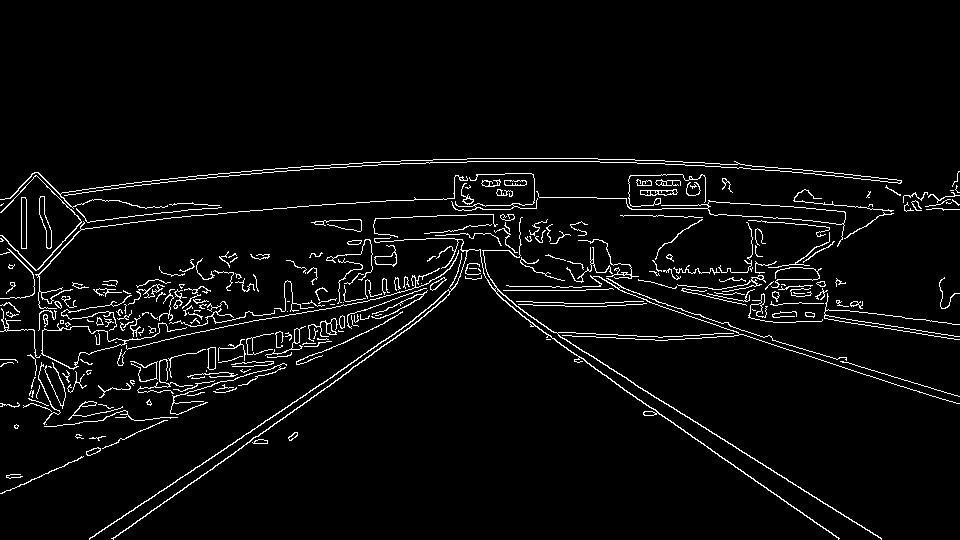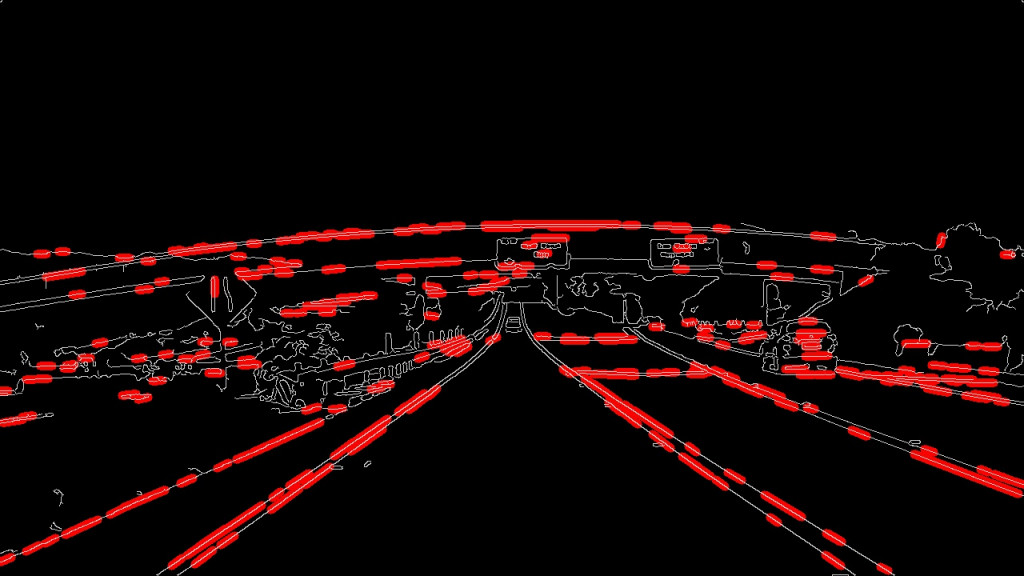#2019 iT 邦幫忙鐵人賽DAY 4
1
AI & Data

# 目的``````lines = cv2.HoughLinesP（masked_edges，rho，theta，threshold，np.array（[]），
min_line_length，max_line_gap）
``````

# 程式執行流程及步驟

## 1.執行import 載入相關函式庫

``````import matplotlib.pyplot as plt
import matplotlib.image as mpimg
import numpy as np
import cv2
``````

## 2.讀入灰階圖像

``````image = mpimg.imread（'exit-ramp.jpg'）
gray = cv2.cvtColor（image，cv2.COLOR_RGB2GRAY）
``````

## 3.定義內核大小並應用高斯平滑處理

``````kernel_size = 5
blur_gray = cv2.GaussianBlur（gray，（kernel_size，kernel_size），0）
``````

## 4.定義Canny的參數並應用

``````low_threshold = 50
high_threshold = 150
masked_edges = cv2.Canny（blur_gray，low_threshold，high_threshold）
``````

## 5.定義Hough轉換參數

``````rho = 1
theta = np.pi/180
threshold = 1
min_line_length = 10
max_line_gap = 1
``````

## 6.製作與我們的圖像大小相同的空白

``````line_image = np.copy(image)*0 #creating a blank to draw lines on
``````

## 7.在邊緣檢測到的圖像上運行霍夫轉換

``````lines = cv2.HoughLinesP（masked_edges，rho，theta，threshold，np.array（[]），
min_line_length，max_line_gap）
``````

## 8.遞廻輸出“lines”並在空白處繪製線條

``````    for x1，y1，x2，y2 in line：
cv2.line（line_image，（X1，Y1），（X2，Y2），（255,0,0），10）
``````

## 9.創建彩色之二進制圖像與線圖像組合

``````color_edges = np.dstack（（masked_edges，masked_edges，masked_edges））
``````

## 10.在邊緣圖像上繪製線條

``````combo = cv2.addWeighted(color_edges, 0.8, line_image, 1, 0)
plt.imshow(combo)
``````0